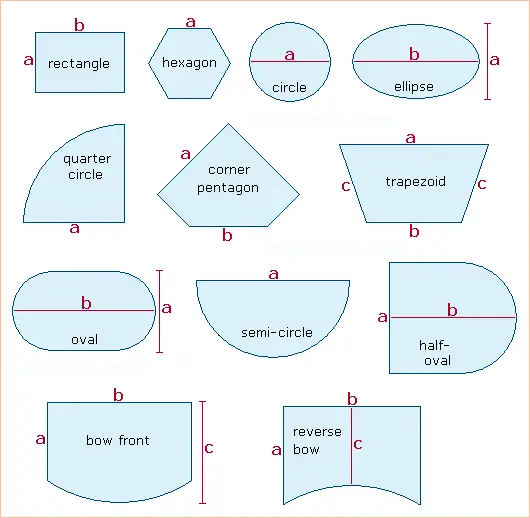# How to Compute the Surface Area and Volume of a Fish Tank

 Fish Tank Calculator Units inches  cm Tank Shape Rectangle Hexagon Circle Ellipse Quarter Circle Corner Pentagon Trapezoid Oval Semi-Circle Half-Oval Bow Front Reverse Bow Dimensions (See chart for positions of a, b, and c. Some may be blank) a = b = c = Tank Height Gravel Type Fine Medium Coarse Gravel Height Tank Capacity (gallons) = Tank Capacity (liters) = Water Surface Area = Glass Surface Area =

The volume and surface area of a fish tank depends on its shape and dimensions. When calculating the total capacity of an aquarium, you must also consider how much water is displaced by the sand and/or gravel at the bottom of the tank.

You can use the calculator on the left and the shape chart below to compute the fish tank's capacity, the surface area of the water, and the surface area of the glass. Select the tank's base shape from the chart and enter the measurements labeled a, b, and c in the calculator. (Some shapes only require values for a and b, some require only a single value for a.) The equations are explained below.

### Calculating Water Surface Area

The amount of oxygen available to your fish is directly proportional to the surface area of the water. The larger the surface area the more fish your aquarium can support. For a rectangular tank, the surface area is width times length. For a circular tank, the surface area is π/4 times the diameter squared (or equivalently, π times the radius squared). If your aquarium's base is a more complicated shape, use the diagram below and the calculator.Always measure inside the tank. If you measure along the outside, your measurements will include the thickness of the glass, which will make your final calculations too large.

### Calculating Aquarium Volume

Your tank's capacity is the main limiting factor in how many fish you can keep. To calculate the volume of an empty aquarium, multiply the water surface area by the height. Again, measure the height from the inside of the tank so that you do not include the thickness of the base.

To figure the capacity of your fish tank when it is filled with rocks or gravel, you must subtract the volume of water displaced by the rocks. The displacement volume depends on the height of the gravel layer, the surface area of the base, and the coarseness of the material. If the area of the base is A and the height of the gravel is H, then the volume of the material is given by one of the three formulas below:

Vol ≈ 0.9HA     fine
Vol ≈ 0.75HA   medium
Vol ≈ 0.6HA     coarse

### Calculating the Surface Area of the Glass

Algae grows on the surface of the glass inside the aquarium. Knowing the surface area of the glass will help you predict and control algae growth, so your fish can thrive. The area of the glass is equal to the inside perimeter of the tank times the height.

For a rectangular tank, the perimeter is 2(W+L), where W and L are the width and length. For a circular tank, the perimeter is π times the diameter, or 2π times the radius.